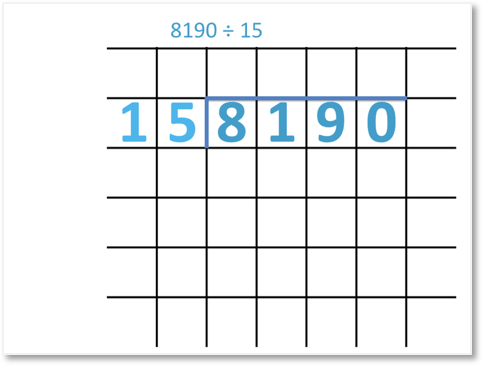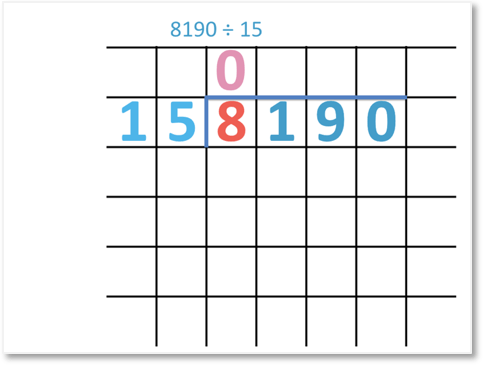# Long Division Method: Divide Large Numbers by 2-Digit Numbers

Long Division Method: Divide Large Numbers by 2-Digit Numbers

Supporting Lessons

## How to use the Long Division Method to Divide Larger Numbers by 2-Digit Numbers

To divide large numbers using the long division method, we set out the calculation as shown below.We will divide the digits of 8190 by 15.15 doesn’t divide into 8, so we will divide 81 by 15.To find out how many times 15 divides into 81, we can list some of the numbers in the 15 times table.

We can stop when we have gone past 81.

75 is the closest to 81 without gong past it.

15 x 5 = 75

So, 81 ÷ 15 = 5

We write the 5 above the 1.

To find the remainder of our division, we subtract 75 from 81.81 – 75 = 6

The remainder is 6.

We bring down the 9 and continue with the long division method.Next, we find out how many times 15 divides into 69.

We can see that 4 x 15 = 60. So, 15 divides into 69 four times.

To find the remainder, we subtract 60 from 69.69 – 60 = 9

The remainder is 9.

We bring down the 0 and continue with the long division method.From the 15 times table that we have written, we can see that 90 ÷ 15 = 6.

Therefore,

8190 ÷ 15 = 546